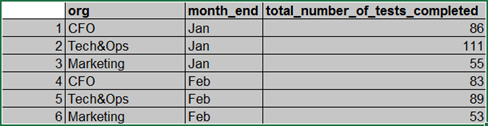# Group ordering in a multi-level vbar plot

hi,

I have a vbar plot that I’m trying to layout by 2 different groups on the xaxis. I’m using a pandas dataframe to read an Excel file with the data.
My outer group is ‘month_end’ and is the names of different months. when I display the plot, the outer group is ordered alphabetically so that “Feb” shows before “Jan”.
My question is, can I use a pandas dataframe and a ColumnDataSource and still order the outer group by the order in which the months are chronologically sequenced? Jan, Feb, March, etc.

``````df = pd.read_excel('org_compliance_timeseries.xlsx', index_col=0)
df.org = df.org.astype(str)
df.month_end = df.month_end.astype(str)
dfgroup = df.groupby(by=['month_end', 'org'])
source = ColumnDataSource(dfgroup)

# periods = ['Jan', 'Feb']
index_cmap = factor_cmap('month_end_org', palette=OrRd3, factors=sorted(df.month_end.unique()), end=1)

p = figure(title="org by month",
x_range=dfgroup, toolbar_location=None, tooltips=[("Tests Completed", "@total_number_of_tests_completed_mean"), ("month_end, org", "@month_end_org")], plot_width=600)
p.vbar(x='month_end_org', top='total_number_of_tests_completed_mean', width=0.9, source=df2group, line_color="black", fill_color=index_cmap)
``````Yes, just change `x_range=dfgroup` to an explicit list of months.
This is the code in Bokeh that does the sorting:

``````[...]
if pd and isinstance(range_input, pd.core.groupby.GroupBy):
return FactorRange(factors=sorted(list(range_input.groups.keys())))
[...]
``````

When using `figure`, you don’t have to call `FactorRange` yourself, just pass a list like `x_range=['Jan', 'Feb', ...]`.

thanks, I modified my code to:

``````    index_cmap = factor_cmap('month_end_cio', palette=GnBu3, factors=df2.month_end.unique(), end=1)

p = figure(title="Org by Period",
x_range=['Jan', 'Feb'], toolbar_location=None, tooltips=[("Tests Completed", "@total_number_of_tests_completed_mean"), ("month_end, org", "@month_end_org")], plot_width=600)
p.vbar(x='month_end_org', top='total_number_of_tests_completed_mean', width=0.9, source=dfgroup, line_color="black", fill_color=index_cmap)
``````

but the plot does not appear to display anymore, and there is no error message (which were displayed before the change) other than:

``````WARNING:bokeh.core.validation.check:W-1005 (FIXED_SIZING_MODE): 'fixed' sizing mode requires width and height to be set: Column(id='1545', ...)
WARNING:bokeh.core.validation.check:W-1005 (FIXED_SIZING_MODE): 'fixed' sizing mode requires width and height to be set: Row(id='1541', ...)
WARNING:bokeh.core.validation.check:W-1005 (FIXED_SIZING_MODE): 'fixed' sizing mode requires width and height to be set: Row(id='1542', ...)
WARNING:bokeh.core.validation.check:W-1005 (FIXED_SIZING_MODE): 'fixed' sizing mode requires width and height to be set: Row(id='1543', ...)
WARNING:bokeh.core.validation.check:W-1005 (FIXED_SIZING_MODE): 'fixed' sizing mode requires width and height to be set: Row(id='1544', ...)
``````

if I go back to using
p = figure(title=“org by month”,
x_range=dfgroup, toolbar_location=None, tooltips=[(“Tests Completed”, “@total_number_of_tests_completed_mean”), (“month_end, org”, “@month_end_org”)], plot_width=600)
the plot does display, but with alphabetically sorted months.

Ah, right - you have two-level factors. Just specify tuples then instead of months’ names, like `x_range=[('Jan', 'CFO'), ('Jan', 'Marketing'), ...]`.
You can follow the example here: https://docs.bokeh.org/en/latest/docs/user_guide/categorical.html#nested-categories

thanks! was able to recreate following the example above.

2 Likes